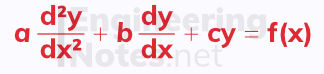top of page

#### Notes by Category University Engineering

Rate these notesNot a fanNot so goodGoodVery goodBrillRate these notes

# Differential Equations

In single maths, first-order differential equations are the only ones looked at, and are solved by separating the variables:

When dy/dx = f(x) g(y), you can say ∫ 1/g(y) dy = ∫ f(x) dx
• Move all the y terms to the left where the dy is

• Move all the x terms to the right, including the dx

This allows you to integrate each side with respect to the variable on that side to solve the equation.

• You only need to add the ' +c ' to one side.Just like when integrating an indefinite function, the initial result is a general solution and could be anywhere along the y-axis (see section on indefinite integral functions above). To fond the particular solution, you need to know a coordinate point on the curve - sometimes this is called a boundary condition.

### Integrating Factor

This is not covered in single maths, but is an important method of solving first-order differential equations where x and terms are multiplied by one another in one of the terms:1. Rearrange to be in the form dy/dx + P(x)y = Q(x)

2. Find the integrating factor using the formula above

3. Multiply the dy/dx by the integrating factor and by y

4. Multiply the right hand side by the integrating factor & simplify (if you can)

5. The middle term, P(x)y disappears

6. Move the dx to the right hand side and integrate this side

7. Rearrange to make y the subject - this is the general solution

8. Find the particular solution by substituting in boundary conditions (if there are any)At further stage, we also look at second-order differential equations - these come in two types: homogenous and non-homogenous.

## Second-Order Homogeneous Differential Equations

Second-order homogeneous differential equations can be solved when in the form:Homogeneous means it equals zero

The solution in terms of y will have four constants that need to be found:

• λ and μ, which are found by solving the auxiliary equation

• A and B, which can only be found if you have boundary conditions

The auxiliary equation is am² + bm + c = 0, and its solutions are λ and μ
• a, b, and c are the coefficients in the second-order homogenous differential equation above.

The format of the solution in terms of y depends on the auxiliary equation:## Second-Order Non-Homogeneous Differential Equations

Second-order homogeneous differential equations can be solved when in the form:• The left hand side of this equation is known as the corresponding homogeneous equation and its solution is called the complementary function.

• The right hand side of this equation is a function of x and its solution is called the particular integral.

Non-homogeneous means the corresponding homogeneous equation equals a function of x

To solve a second-order non-homogeneous differential equation, first solve the corresponding homogeneous equation as normal (see above), and then find the particular integral:

1. Take the correct standard function

2. Find dy/dx and d²y/dx²

3. Substitute these into the initial equation

4. Solve to find the constants in the standard function

The form of the standard function depends on the form of f(x):When the particular integral is already in the complementary function, you need to multiply the particular integral by x

For example, if f(x) is in the exponential form where k is the same as one of the roots in the auxiliary equation, multiply the particular integral by x.